In this post we will use Matlab to filter angular velocity data acquired with an Arduino. The gyroscope we will be using is a tri-axis L3G4200D sensor. In a previous post – Can you roll with a L3G4200D gyroscope, Arduino and Matlab? we have seen how to configure it and extract the angular rates using the i2c interface.

The chip costs 5\$ and you can find the breakout board around 50\$.

The angular rates are used as an input of a nonlinear controller in a quadcopter application. We will assume that we are not interested in removing the bias from the readings as we have already discussed it in Filtering Gyroscope data with Matlab.

When the sensor is steady, the plot shows several undesired spikes.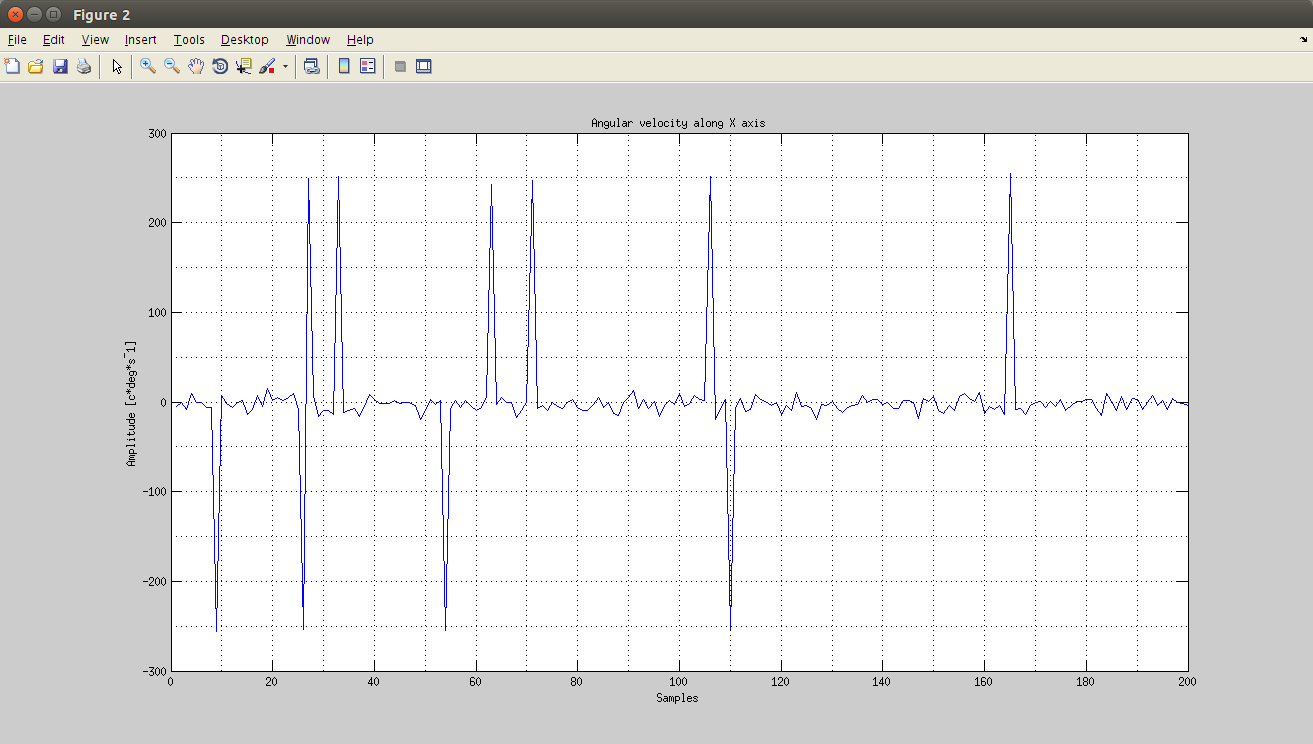The aim of this post is to suppress these spikes. Please comment and provide suggestions thanks!

### First Approach: ButterWorth low pass filter

In a quadcopter application, you will encounter different types of angular rate signals. For example, we would like to implement a control strategy that compensates undesired tilt of a given angle. In this situation, the angular velocity along the considered axis will increase. That’s the signal’s component we want to preserve. Let’s consider the following samples, acquired at 200Hz, in which a couple of fast rotations are performed. Let’s assume that the frequency components of the “fast movement” are the ones I will deal with in the final application.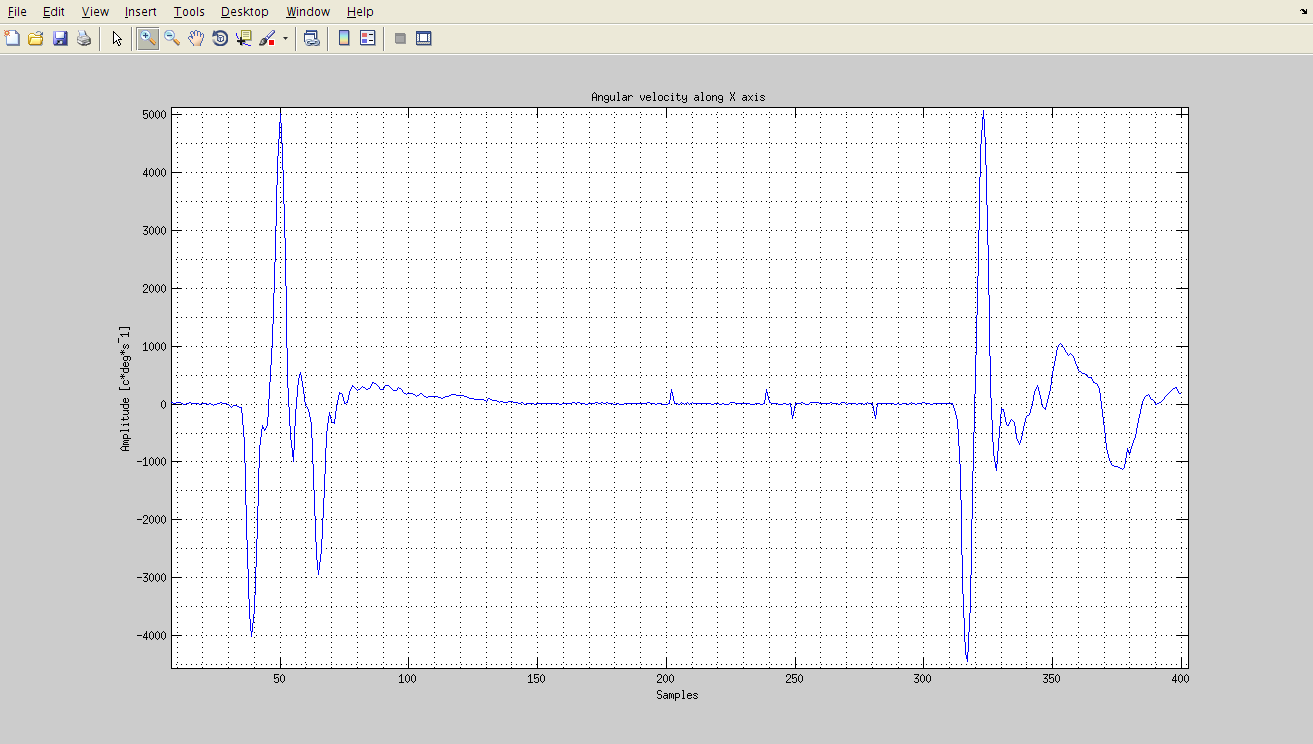By analyzing the signal in the frequency domain we can recognize the frequency components of the “fast rotation” we would like to keep track of.
Below, the discrete Fourier transform of the signal in a normalized frequency scale and the candidate second order ButterWorth low pass filter.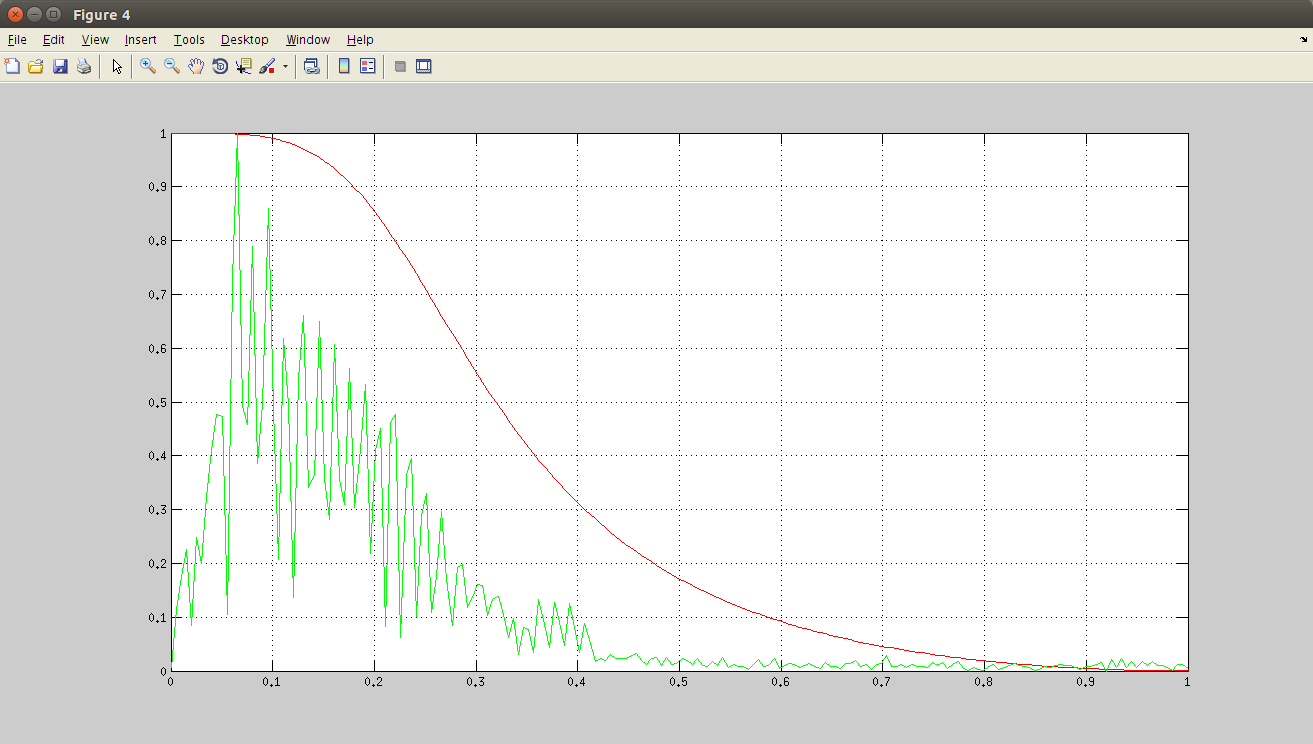The filtered signal is shown in the next image. As you can see, we should try to leave the main spikes unaffected and attenuate the small ones (from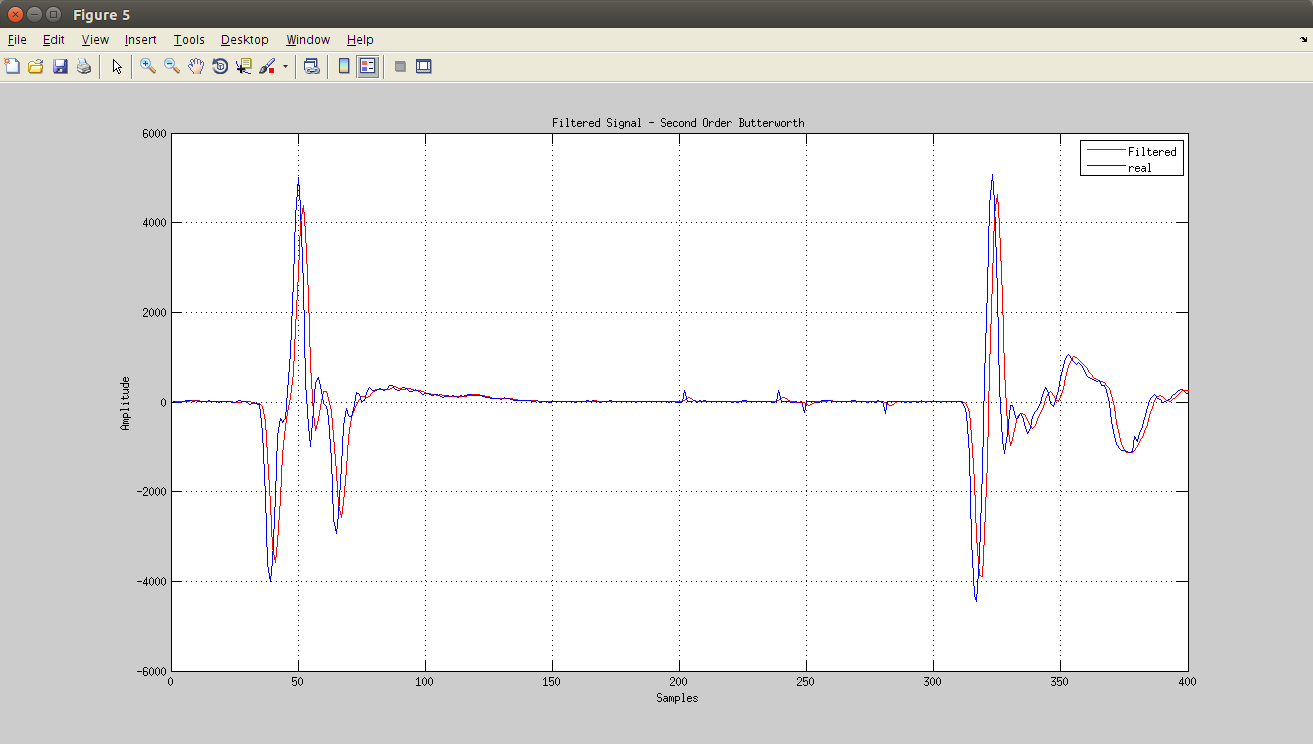The undesired spikes are attenuated but still present in the signal.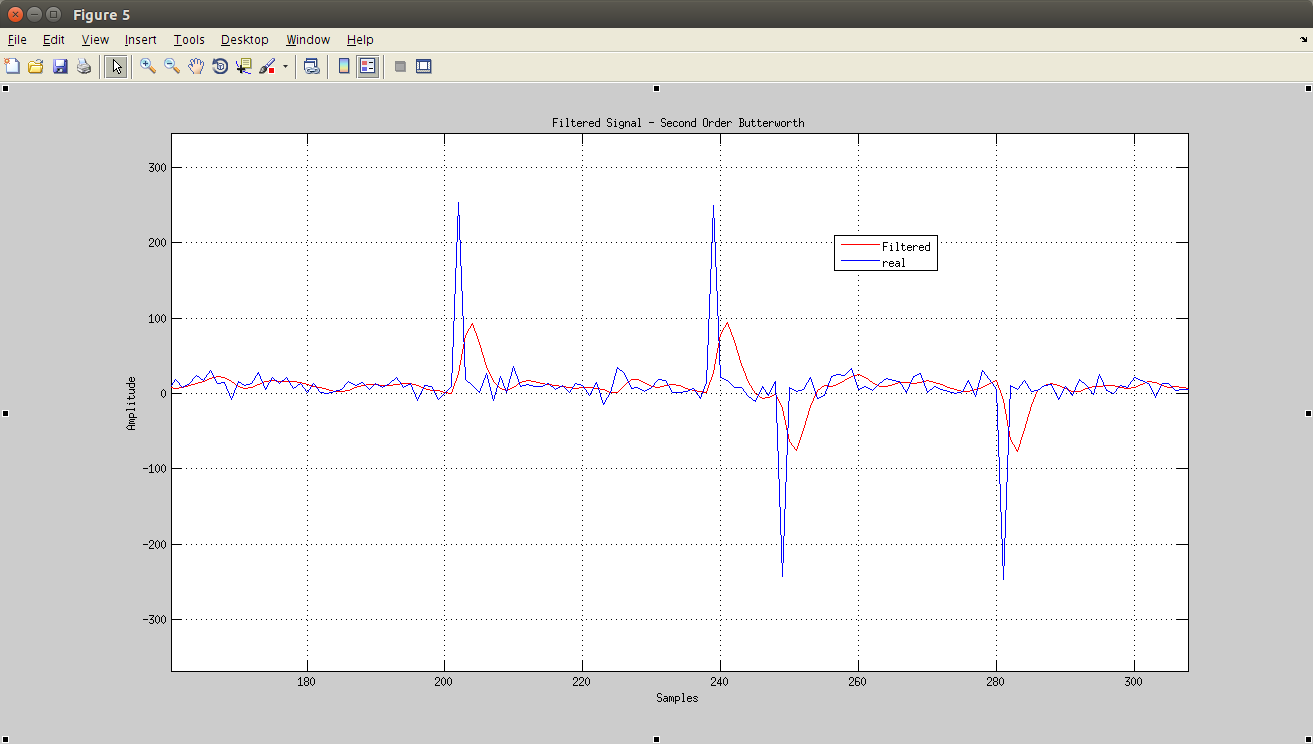The solution

The undesired spikes can be removed using a Median Filter. Soon a few screenshots of the filtered signal.

Please comment this post and suggest other techniques to filter the data.

Thanks to: